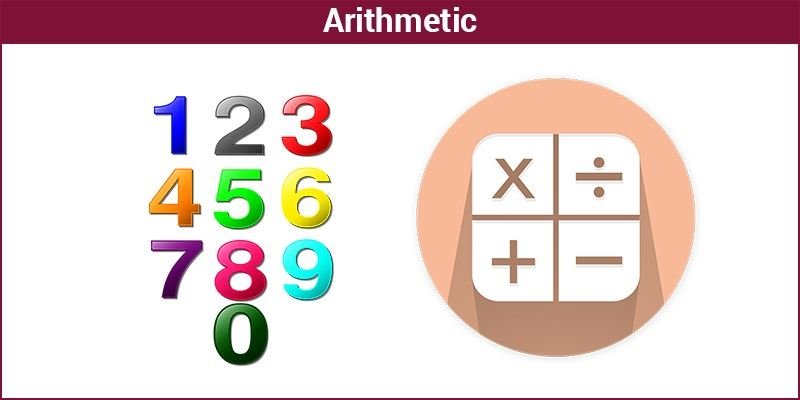# Arithmetic

Arithmetic is one among the oldest and elementary branches of mathematics, originating from the Greek word arithmos, means number. It involves the study of numbers, especially the properties of traditional operations on them such as addition, subtraction, division and multiplication.

These are the basic operations, although the subject also involves advanced operations like computation of percentages, logarithmic functions, exponentiation and square roots.

## History of Arithmetic

The Fundamental principle of number theory was provided by Carl Friedrich Gauss in 1801, according to which, any integer which is greater than 1 can be described as the product of prime numbers in only one way. Arithmetic is another name given to number theory. The four elementary operations in arithmetic are addition, subtraction, multiplication and division. All these operations we are going to discuss in brief here.## Arithmetic operations

The basic operations under arithmetic are addition and subtraction, division and multiplication although the subject involves many other modified operations.

Addition is among the basic operations in arithmetic. In simple forms, addition combines two or more values into a single term, for example: 2 + 5 = 7, 6 + 2 = 8.

The procedure of adding more than two values is called summation and involves methods to add n number of values.

The identity element of addition is 0, which means that adding 0 to any value gives the same result. The inverse element of addition is the opposite of any value, which means that adding opposite of any digit to the digit itself gives the additive identity. For instance, the opposite of 5 is -5, therefore 5 + (-5) = 0.

### Subtraction (−)

Subtraction can be labelled as the inverse of addition. It computes the difference between two values, i.e, the minuend minus the subtrahend. If the minuend is greater than the subtrahend, the difference is positive. If the minuend is less than the subtrahend, the result is negative, and 0 if the numbers are equal.

### Multiplication (×)

Multiplication also combines two values like addition and subtraction into a single value or the product. The two original values are known as the multiplicand and the multiplier, or simply both as factors.

The product of a and b is expressed as a·b or a x b. In software languages wherein only characters are used that are found in keyboards, it is often expressed as, a*b (* is called asterisk).

### Division (÷)

Division is the inverse of multiplication. It computes the quotient of two numbers, the dividend that is divided by the divisor. The quotient is more than 1 if the dividend is greater than divisor for any well-defined positive number, else it is smaller than 1.

### Arithmetic Problems

Question 1: The sum of two numbers is 50 and their difference is 30. Find the numbers.

Solution: Let the numbers be x and y. Now, as per the given situation,

x + y = 50……………………(i)

and x – y = 30………………(ii)

We can write, x = 50-y, from eq.(i),

Therefore, putting the value of x in eq(ii), we get,

50 – y – y = 30

50 -2y = 30

2y = 50-30= 20

y = 20/2 = 10

and x = 50 – y = 50-10 =40

Therefore, the two numbers are 40 and 10.

Question 2: Solve 25+5(27÷3)-9

Solution: 25 + 5(27 ÷ 3) – 9

⇒  25 + 5(9) – 9

⇒ 25 + 45 – 9

⇒ 70 – 9 = 61

### What is Arithmetic in Maths?

Arithmetic is one of the branches of mathematics which deals with different types of number like odd numbers, whole numbers, even numbers, etc. and their basic operations involving addition, subtraction, multiplication, and division.

### What are the Properties of Operation in Arithmetic?

There are four main properties of operations which include:

• Commutative Property
• Associative Property
• Distributive Property

### What is the Oder of Operations?

The BODMAS or PEMDAS rule is followed to for order of any operation involving +, −,×, and ÷. The order of operation is:

B: Brackets

O: Order

D: Division

M: Multiplication